# Variance Calculator

To find the variance, select the sample or population option, enter the comma-separated values, and click caluclate button using variance calculator

Give Us Feedback

## Variance Calculator with steps

Variance calculator is used to find the variance of sample and population data. This variance solver takes the comma-separated values and gives the result of variance, standard deviation, the mean, and the statistical sum of squares in one click.

## What is a variance?

In statistics, the average of the squared deviations from the mean is said to be the variance. It decides whether the data values are closer or far from the average value.

The small variance tells that the random data values are closer to the mean. While the greater variance indicates that the random data values are far from the mean.

### Types of variances

1. Sample variance
2. Population variance

## Formulas of variance

The formula for the population variance is: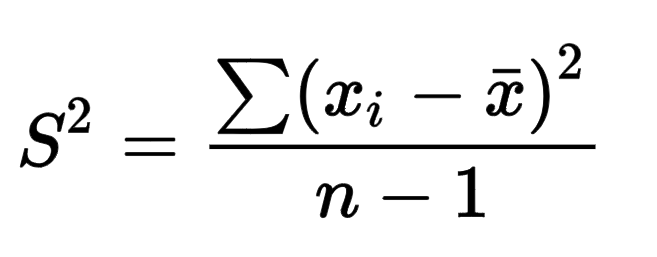The formula for the sample variance is: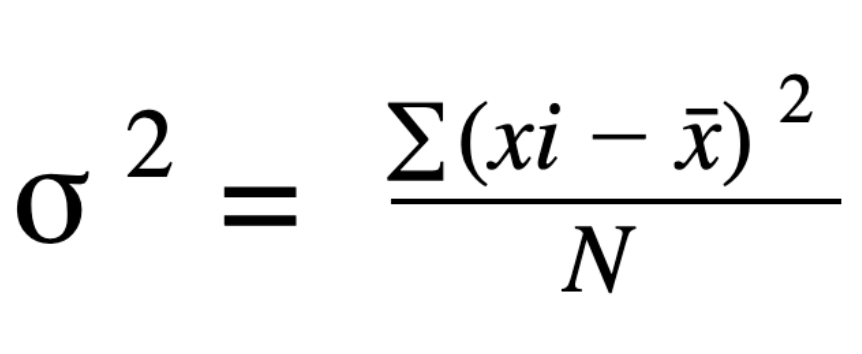## How to calculate variance?

Follow the below examples to learn how to calculate the variance.

Example 1: For sample variance

Find the sample variance of 12, 14, 15, 19, 25?

Solution

Step 1: First of all, calculate the mean of sample data.

Mean = x̅ = Σx/n

= [12 + 14 + 15 + 19 + 25]/5

= 85/5

= 17

Step 2: Now find the difference of each data value from the mean and the square of the differences.

 Data values (x) xi - x̅ (xi - x̅)2 12 12 – 17 = -5 (-5)2 = 25 14 14 – 17 = -3 (-3)2 = 9 15 15 – 17 = -2 (-2)2 = 4 19 19 – 17 = 2 (2)2 = 4 25 25 – 17 = 8 (8)2 = 64

Step 3: Find the statistical sum of squares.

Σ(xi - x̅)= 25 + 9 + 4 + 4 + 64

=  106

Step 4: Take the formula of sample variance and substitute the values.

Σ(xi - x̅)2 / n-1 = 106/5-1

= 106/4

= 26.5

Try the sample variance calculator above to check the accuracy of steps and result.

Example 2: For population variance

Find the population variance of 10, 24, 29, 35, 36, 40.

Solution

You can solve this problem by using the population variance calculator above or manually.

Manually

Below are the steps to solve this problem manually.

Step 1: First of all, calculate the mean of population data.

Mean = µ = Σx/n

= [10 + 24 + 29 + 35 + 36 + 40]/5

= 174/6

= 29

Step 2: Now find the difference of each data value from the mean and the square of the differences.

 Data values (x) xi - µ (xi - µ)2 10 10 – 29 = -19 (-19)2 = 361 24 24 – 29 = -5 (-5)2 = 25 29 29 – 29 = 0 (0)2 = 0 35 35 – 29 = 6 (6)2 = 36 36 36 – 29 = 7 (7)2 = 49 40 40 – 29 = 11 (11)2 = 121

Step 3: Find the statistical sum of squares.

Σ(xi - µ)2 = 361 + 25 + 0 + 36 + 49 + 121

= 592

Step 4: Take the formula of sample variance and substitute the values.

Σ(xi - µ)2/n = 106/6

= 98.667

Using calculator

Using the population variance calculator above.

Step 1: Select the type of variance.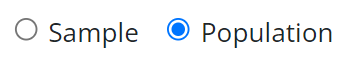Step 2: Enter the comma-separated values.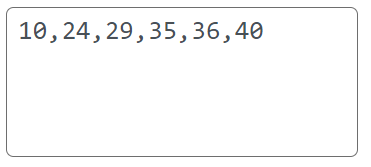Step 3: Click calculate buttonThe result will come in a fraction of a second.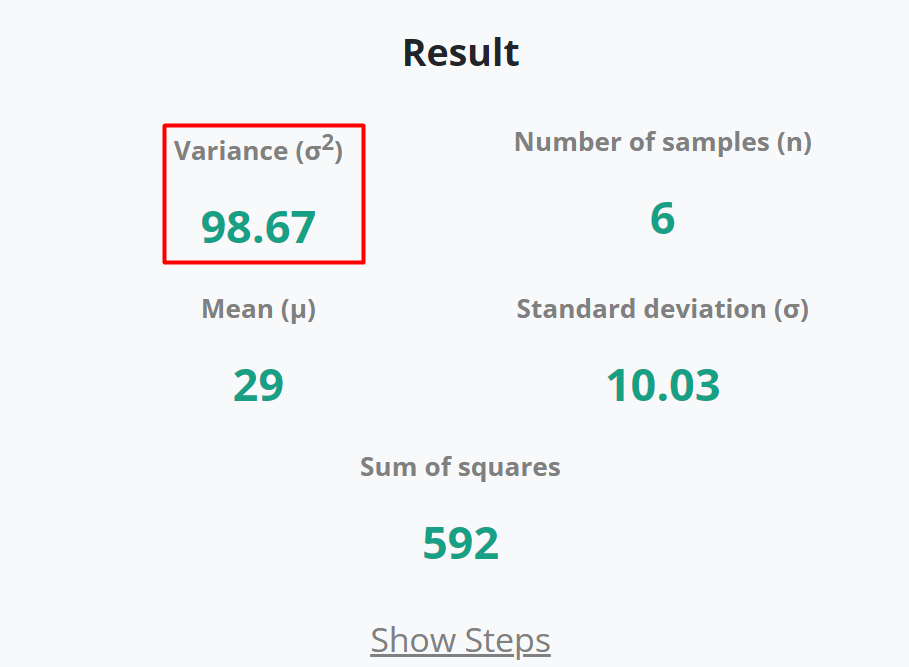Step 4: Press show steps to view the step-by-step calculations of variance.Step-by-step solution for variance calculations will appears as: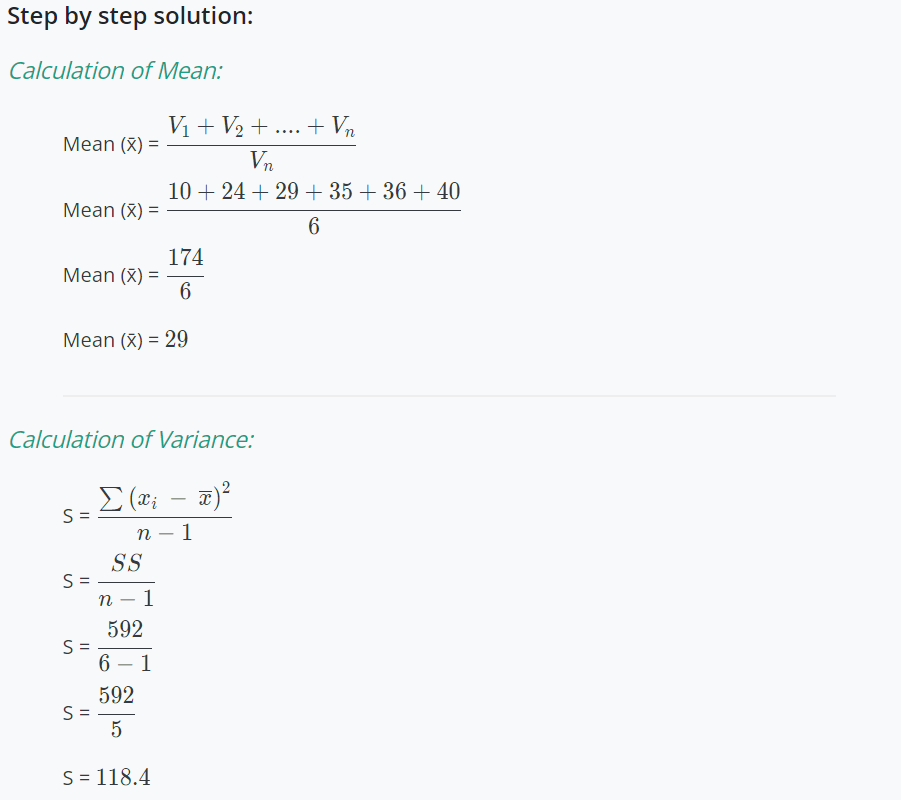### Math Tools